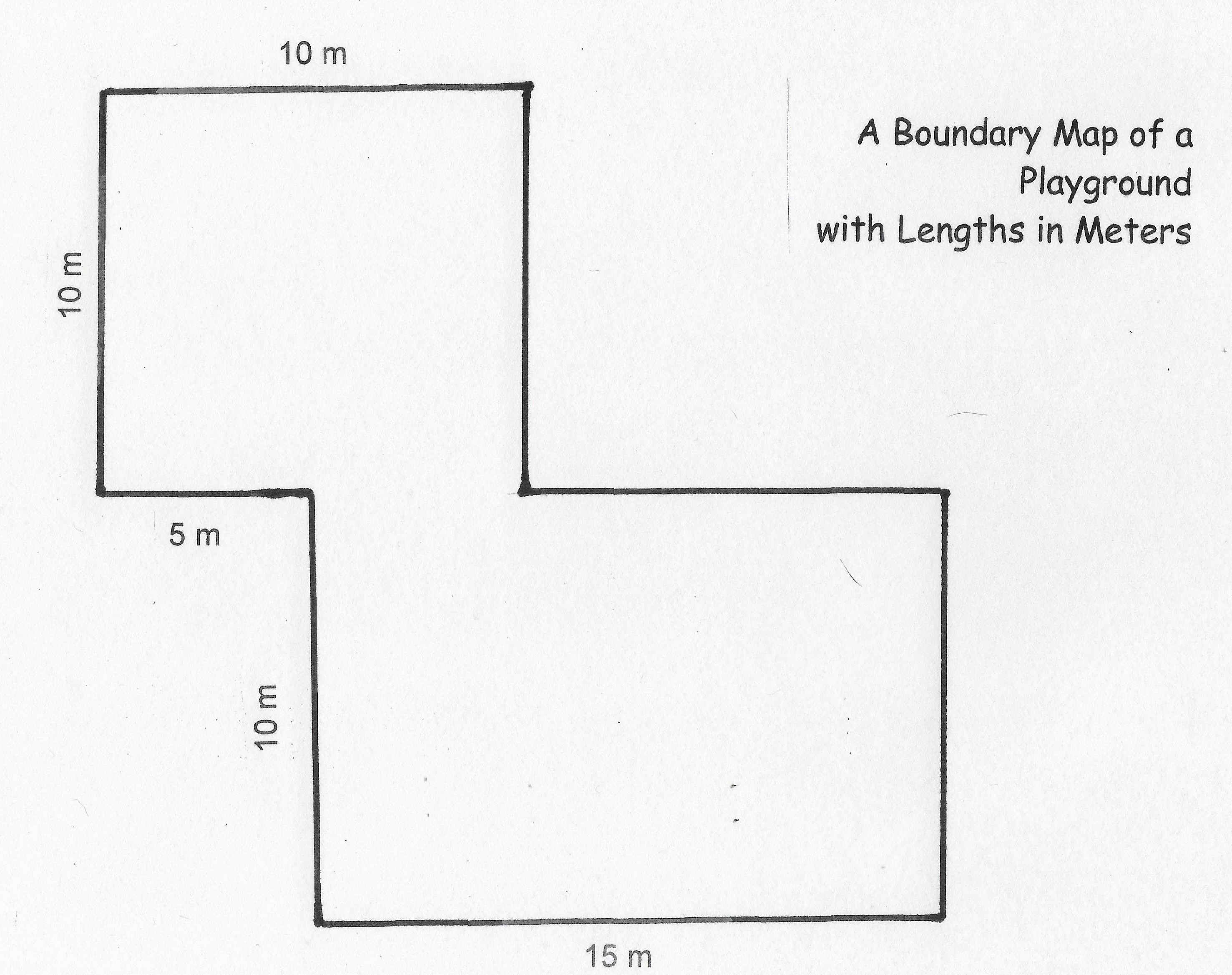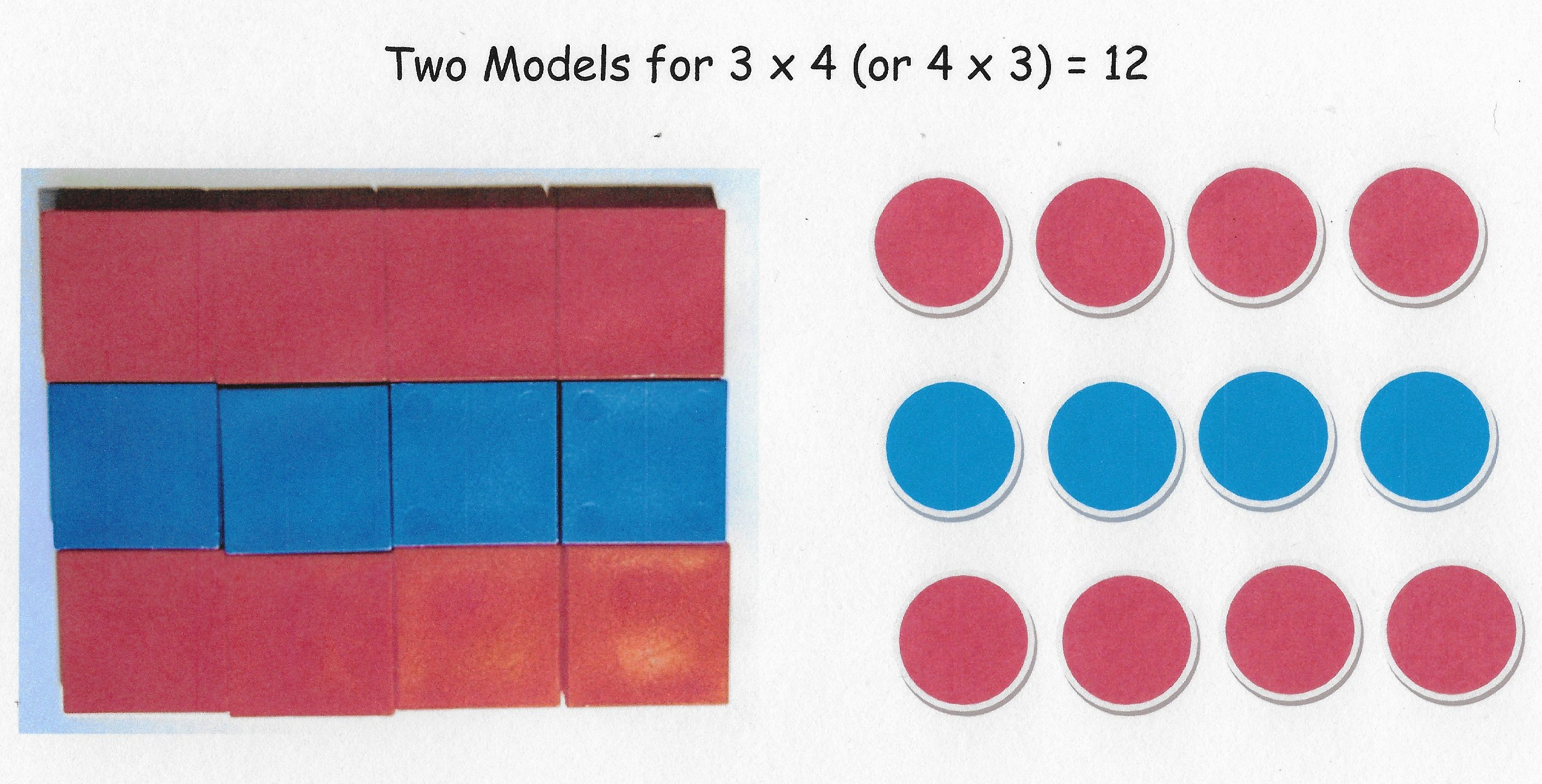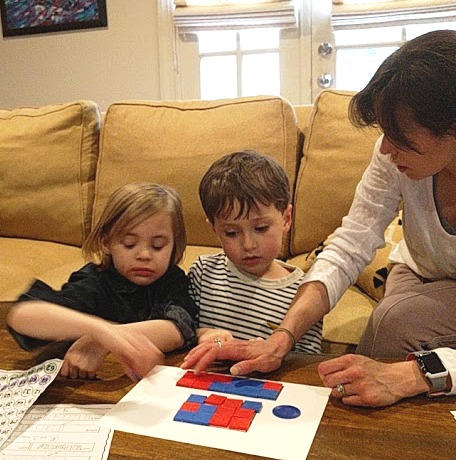# Area of a Playground

A map indicating the shape and dimensions of a small, neighborhood playground is shown below.  Find the area of the playground in square meters.To answer this test question correctly, a student is expected to understand the concept of area (Goal # 9) and compose two shapes mentally into one region.  By adding the area of the square section (100 square meters) to the rectangular section (150 square meters) we find the playground’s area to be 250 square meters.   An understanding of place value (Goal #3) is also needed to determine mentally that the product of 15  and 10  is 150.

Area is the amount of a 2-dimensional surface that is contained within a boundary, a complex yet extremely important measurement concept.  We can begin talking about basics of area with prekindergarten children, and these conversations can lead to proficiency by third grade, future understandings in calculus, as well as the idea of a distribution in statistics. Here are some ways to introduce the idea of area.

• A large table top will have more space to place things on top of it than a smaller one; and a larger plate will hold more cookies.
• Begin making informal area measurements by filling your bounded surface with beans, packing peanuts or other countable material.  Talk about how it takes more measuring units to cover a larger space than a smaller space and about the same amount of measuring units to cover spaces of the same size.
• Two-dimensional shapes can be decomposed and composed, and the total area remains the same.  The total area of a completed puzzle’s surface is the sum of the areas of each piece.   Show a young child how a piece of paper fits back together as a whole after tearing  it apart.### Connecting Area of Rectangles with Row-Column Arrays

Area is a powerful model for understanding multiplication and division and learning the multiplication facts from memory (Goal #2).   To help a child visualize multiplication facts before they are memorized,  represent them in multiple ways. For example, relate the fact 3 x 4 = 12 to both a 3-inch by 4-inch rectangle filled with 12 one-inch square tiles and a rectangular array of dots with 3 rows and 4 columns.Another thing about area is that rectangles of different shapes can have the same area.   In this photo, the children are tiling one 3-inch by 4-inch rectangle and another that is 6 inches by 2 inches.  Upon observing, the children can see that the two rectangles are different shapes –one longand thin, the other wider and closer to being square.  However, when they count the tiles in the two rectangles they will find both rectangles cover a 12- square-inch area.

In closing, note that Power-Start Goal #9 mentions understanding of perimeter as well as area as a third-grade expectation.    In next week’s  test question,  we’ll consider a problem about both the perimeter and area of a playground.   Unlike the square inches or square yards used to measure area, perimeter is measured with a ruler or measuring tape in feet, meters, etc.   Furthermore, shapes with the same area can have different perimeters.